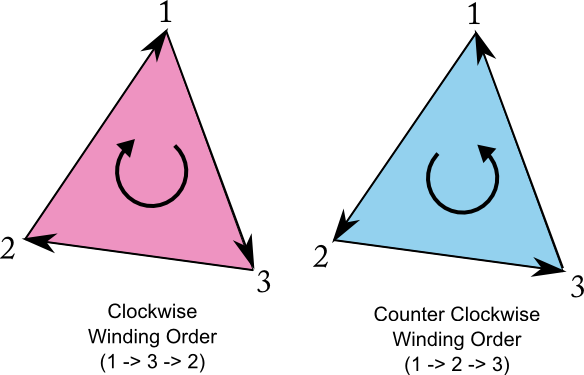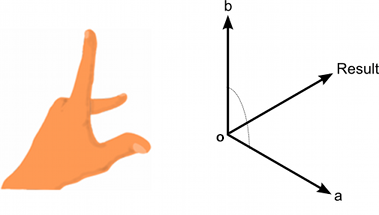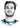# Understanding front faces - winding order and normals

Categories:

Whether a side of a face is considered to be the front or the back, depends on the winding order the game engine uses.

### Winding Order

Consider you have a triangle (the same idea works for any polygon) and you start by picking any vertex (call this vertex 1). Then you can pick up the other vertices from your point of view in either a clockwise or counter-clockwise order. The way you do this is called the winding order of the triangle.

In the image below the order 1,3,2 would be a clockwise (CW) winding order; 1,2,3 would be counter-clockwise (CCW). If you change your point of view to look at the triangle from the other side, you would perceive the opposite winding order, 1,3,2 would be CCW, 1,2,3 CW.Now you define a face following a certain winding order - say CCW - to be the front face, the other side to be the back face. Which winding order corresponds to the front is completely arbitrary and depends on the game engine you use. However for some reason this fact is seldom documented and you have to find out yourself through testing. Unity uses a clockwise winding order for front faces. Blender exports in a counter-clockwise winding order.

### What about the normal of the face?

The normal has nothing to do with determining the front/back of a face. There is (unfortunately) no rule that says that the normal always has to face towards the front. However, it would make sense as the normal is usually used for determinig the amount of light the face will receive. For example in the Phong Illumination Model the more similiar the normal N is to the vector L, going from the face to the light, the brighter it will be.

You can calculate “the” normal of a triangle by taking the cross product of its first edge u (1 -> 2) and second edge v (1->3). The reason why I put “the” in quotes is because normals can not only have different magnitudes (let’s normalize it to have unit length for now), but more severe its direction is not unique. Reflecting the normal along the face will yield another normal with a different direction. Let’s say we want the normal to face in the front direction, whatever that means, depending on the winding order your engine uses. Then the cross product of u and v yields the normal according to the Right-Hand-Rule. If you look up Unity’s documentation for Vector3.Cross, you see Unity uses a left-hand-coordinate system, so the direction will be according to the Left-Hand-Rule.Left Hand rule for determining the direction of Unity’s Vector3.Cross(a,b). Index finger points in the direction of a, middle finger points towards v, the resulting vector a x b has the direction of your thumb.

Here is some code you can use in Unity that will yield a front-facing normal, depending on the winding order of the mesh you provide.

``````public void Triangle(Vector3 v0, Vector3 v1, Vector3 v2, bool clockwise = true)
{
Vector3 u = v1 - v0;    // edge v0 -> v1
Vector3 v = v2 - v0;    // edge v0 -> v2

// Unity uses Clockwise winding order to determine front-facing triangles
// Unity uses a left-handed coordinate system
// the normal faces front
Vector3 normal = (clockwise ? 1 : -1) * Vector3.Cross(u, v).normalized;
}``````

# Hi, I'm Christoph Michel 👋I'm a , , and .

I mostly do and help small to mid-sized teams from all over the world 🌎 on both short and long-term projects on an independent contractor basis.

I strive for efficiency ⏱️ and therefore track many aspects of my life.